### D.31 In­te­gral Schrö­din­ger equa­tion

In this note, the in­te­gral Schrö­din­ger equa­tion is de­rived from the par­tial dif­fer­en­tial equa­tion ver­sion.

First the time-in­de­pen­dent Schrö­din­ger equa­tion is rewrit­ten in the form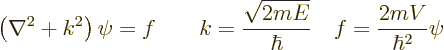(D.16)

The left equa­tion is known as the “Helmholtz equa­tion.”

The Helmholtz equa­tion is not at all spe­cific to quan­tum me­chan­ics. In gen­eral it de­scribes ba­sic wave prop­a­ga­tion at a fre­quency re­lated to the value of the con­stant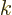. The right hand sidede­scribes the amount of wave mo­tion that is cre­ated at a given lo­ca­tion. Quan­tum me­chan­ics is some­what weird in thatin­volves the un­known wave func­tion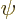that you want to find. In sim­pler ap­pli­ca­tions,is a given func­tion.

The gen­eral so­lu­tion to the Helmholtz equa­tion can be writ­ten as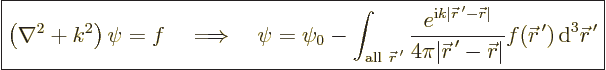(D.17)

Here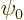is any so­lu­tion of the ho­mo­ge­neous Helmholtz equa­tion, the equa­tion with­out.

To see why this is the so­lu­tion of the Helmholtz equa­tion re­quires a bit of work. First con­sider the so­lu­tion of the Helmholtz equa­tion for the spe­cial case thatis a delta func­tion at the ori­gin: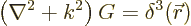The so­lu­tion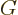to this prob­lem is called the “Green’s func­tion of the Helmholtz equa­tion.

The Green’s func­tion can be found rel­a­tively eas­ily. Away from the ori­ginis a so­lu­tion of the ho­mo­ge­neous Helmholtz equa­tion, be­cause the delta func­tion is every­where zero ex­cept at the ori­gin. In terms of quan­tum me­chan­ics, the ho­mo­ge­neous Helmholtz equa­tion means a par­ti­cle in free space,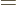0. Pos­si­ble so­lu­tions forare then spher­i­cal har­mon­ics times spher­i­cal Han­kel func­tions of the first and sec­ond kinds, {A.6}. How­ever, Han­kel func­tions of the first kind are pre­ferred for phys­i­cal rea­sons; they de­scribe waves that prop­a­gate away from the re­gion of wave gen­er­a­tion to in­fin­ity. Han­kel func­tions of the sec­ond kind de­scribe waves that come in from in­fin­ity. In­com­ing waves, if any, are usu­ally much more con­ve­niently de­scribed us­ing the ho­mo­ge­neous so­lu­tion.

Fur­ther, since the prob­lem foris spher­i­cally sym­met­ric, the so­lu­tion should not de­pend on the an­gu­lar lo­ca­tion. The spher­i­cal har­monic must be the con­stant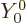. That makes the cor­rect so­lu­tion a mul­ti­ple of the spher­i­cal Han­kel func­tion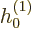, which means pro­por­tional to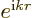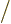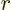. You can eas­ily check by di­rect sub­sti­tu­tion that this does in­deed sat­isfy the ho­mo­ge­neous Helmholtz equa­tion away from the ori­gin in spher­i­cal co­or­di­nates.

To get the cor­rect con­stant of pro­por­tion­al­ity, in­te­grate the Helmholtz equa­tion forabove over a small sphere around the ori­gin. In the right hand side use the fact that the in­te­gral of a delta func­tion is by de­f­i­n­i­tion equal to 1. In the left hand side, use the di­ver­gence the­o­rem to avoid hav­ing to try to in­te­grate the sin­gu­lar sec­ond or­der de­riv­a­tives ofat the ori­gin. That shows that the com­plete Green’s func­tion is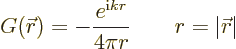(You might worry about the math­e­mat­i­cal jus­ti­fi­ca­tion for these ma­nip­u­la­tions. Sin­gu­lar func­tions likeare not proper so­lu­tions of par­tial dif­fer­en­tial equa­tions. How­ever, the real ob­jec­tive is to find the lim­it­ing so­lu­tionwhen a slightly smoothed delta func­tion be­comes truly sin­gu­lar. The de­scribed ma­nip­u­la­tions are jus­ti­fied in this lim­it­ing process.)

The next step is to solve the Helmholtz equa­tion for an ar­bi­trary right hand side, rather than a delta func­tion. To do so, imag­ine the re­gion sub­di­vided into in­fi­nitely many in­fin­i­tes­i­mal vol­ume el­e­ments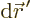. In each vol­ume el­e­ment, ap­prox­i­mate the func­tionby a delta func­tion spike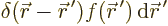. Such a spike in­te­grates to the same value asdoes over the vol­ume el­e­ment. Each spike pro­duces a so­lu­tion given by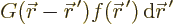In­te­grate over all vol­ume el­e­ments to get the so­lu­tion of the Helmholtz equa­tion (D.17). Sub­sti­tute in whatis for the Schrö­din­ger equa­tion to get the in­te­gral Schrö­din­ger equa­tion.